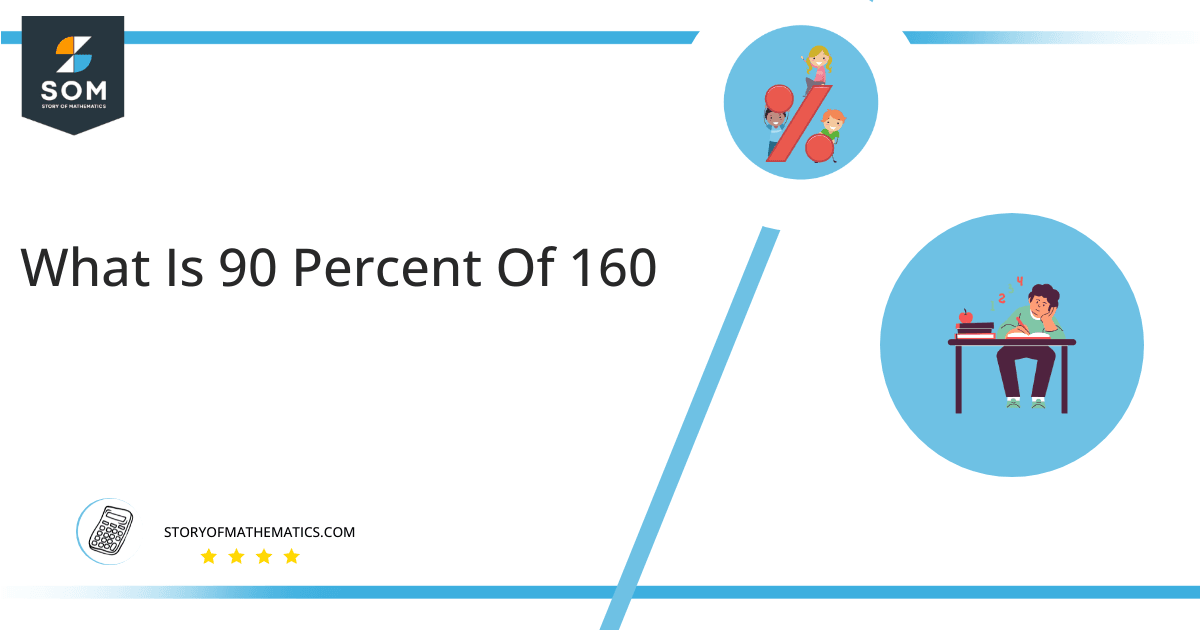# What Is 90 Percent of 160+ Solution with Free Steps?

The 90 percent of 160 is equal to 144. It can be easily calculated by dividing 90 by 100 and multiplying the answer with 160 to get 144.The easiest way to get this answer is by solving a simple mathematical problem of percentage. You need to find 90% of 160 for some sale or real-life problem. Divide 90 by 100, multiply the answer with 160, and get the 90% of 160 value in seconds.

This article will explain the full process of finding any percentage value from any given quantity or number with easy and simple steps.

## What Is 90 percent of 160?

The 90 percent of 160 is 144.

The percentage can be understood with a simple explanation. Take 160, and divide it into 100 equal parts. The 90 number of parts from the total 100 parts is called 90 percent, which is 144 in this example.

## How To Calculate 90 percent of 160?

You can find 90 percent of 160 by some simple mathematical steps explained below.### Step 1

Firstly, depict 90 percent of 160 as a fractional multiple as shown below:

90% x 160

### Step 2

The percentage sign % means percent, equivalent to the fraction of 1/100.

Substituting this value in the above formula:

= (90/100) x 160

### Step 3

Using the algebraic simplification process, we can arithmetically manipulate the above equation as follows:

= (90 x 160) / 100

= 14400 / 100

= 144This percentage can be represented on a pie chart for visualization. Let us suppose that the whole pie chart represents the 160 value. Now, we find 90 percent of 160, which is 144. The area occupied by the 144 value will represent the 90 percent of the total 160 value. The remaining region of the pie chart will represent 10 percent of the total 160 value. The 100% of 160 will cover the whole pie chart as 160 is the total value.

Any given number or quantity can be represented in percentages to better understand the total quantity. The percentage can be considered a quantity that divides any number into hundred equal parts for better representation of large numbers and understanding.

Percentage scaling or normalization is a very simple and convenient method of representing numbers in relative terms. Such notations find wide application in many industrial sectors where the relative proportions are used.